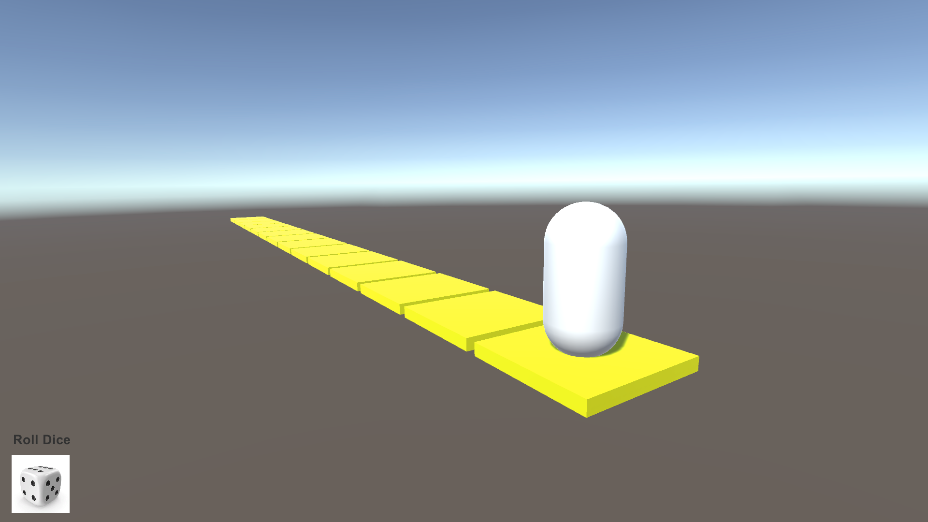# Unity: Create a Simple Board GameRoll a dice and move forward

# Introduction

It’s not difficult to make a simple board game that rolls a dice and move forward. Here is how to do so.

# Prerequisites

1. Basic knowledge of Unity C# programming

# Steps

Step 1: Create a 3D Project

`using System.Collections;using System.Collections.Generic;using UnityEngine;public class GameSceneController : MonoBehaviour{    // Start is called before the first frame update    void Start()    {            }    // Update is called once per frame    void Update()    {            }}`
`using System.Collections;using System.Collections.Generic;using UnityEngine;public class GameSceneController : MonoBehaviour{    private float stepX;    // Start is called before the first frame update    void Start()    {        stepX = 0;    }    // Update is called once per frame    void Update()    {            }    public void RollDice()    {        int result = Random.Range(1, 6);        Debug.Log("Dice rolled: " + result);        stepX += 1.1f * result;    }}`
`using System.Collections;using System.Collections.Generic;using UnityEngine;public class GameSceneController : MonoBehaviour{    public GameObject character;        private float stepX;    private int speed = 3;    // Start is called before the first frame update    void Start()    {        stepX = 0;    }    // Update is called once per frame    void Update()    {        Vector3 target = character.transform.position;        target.x = stepX;        character.transform.position = Vector3.MoveTowards(character.transform.position, target, Time.deltaTime * speed);    }    public void RollDice()    {        int result = Random.Range(1, 6);        Debug.Log("Dice rolled: " + result);        stepX += 1.1f * result;    }}`
1. obtains the position of the character,
2. change the X position to the value of `stepX`
3. Use the `Vector3.MoveTowards()` function to change the character position smoothly.
`using System.Collections;using System.Collections.Generic;using UnityEngine;using UnityEngine.UI;public class GameSceneController : MonoBehaviour{    public GameObject character;    public Sprite dice0;    public Sprite dice1;    public Sprite dice2;    public Sprite dice3;    public Sprite dice4;    public Sprite dice5;    public Sprite dice6;    public Button btnRollDice;    private float stepX;    private int speed = 3;    // Start is called before the first frame update    void Start()    {        stepX = 0;    }    // Update is called once per frame    void Update()    {        Vector3 target = character.transform.position;        target.x = stepX;        character.transform.position = Vector3.MoveTowards(character.transform.position, target, Time.deltaTime * speed);        if(character.transform.position == target)        {            btnRollDice.GetComponent<Image>().sprite = dice0;        }    }    public void RollDice()    {        int result = Random.Range(1, 6);        Debug.Log("Dice rolled: " + result);        stepX -= 1.1f * result;        // Display sprite graphics        switch(result)        {            case 1:                btnRollDice.GetComponent<Image>().sprite = dice1;                break;            case 2:                btnRollDice.GetComponent<Image>().sprite = dice2;                break;            case 3:                btnRollDice.GetComponent<Image>().sprite = dice3;                break;            case 4:                btnRollDice.GetComponent<Image>().sprite = dice4;                break;            case 5:                btnRollDice.GetComponent<Image>().sprite = dice5;                break;            case 6:                btnRollDice.GetComponent<Image>().sprite = dice6;                break;            default:                btnRollDice.GetComponent<Image>().sprite = dice0;                break;        }    }}`